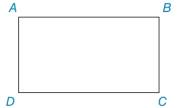Chapter 2.1, Problem 14EElementary Geometry For College St...

7th Edition
Alexander + 2 others
ISBN: 9781337614085

Solutions

Chapter
SectionElementary Geometry For College St...

7th Edition
Alexander + 2 others
ISBN: 9781337614085
Textbook Problem

A D ¯ ∥ B C ¯ , A B ¯ ∥ D C ¯ , and m ∠ A = 92 ° . Find: a) m ∠ B b) m ∠ C c) m ∠ DTo determine

a)

To find:

The find the measure of B.

Explanation

If there exists two parallel lines cut by a transversal then the pair of interior angles on the same side of transversal are supplementary.

Given:

The given figure is,

AD¯BC¯, AB¯DC¯, and mA=92°.

The given angle is A.

Approach:

Consider the given figure,

From the above figure, AD¯BC¯ with transversal AB¯

To determine

b)

To find:

The find the measure of C.

To determine

c)

To find:

The find the measure of D.

Still sussing out bartleby?

Check out a sample textbook solution.

See a sample solution

The Solution to Your Study Problems

Bartleby provides explanations to thousands of textbook problems written by our experts, many with advanced degrees!

Get Started

27. Show that the function is a probability density function.

Mathematical Applications for the Management, Life, and Social Sciences

Solving Inequalities Solve the inequality. 80. 3 log2 x 4

Precalculus: Mathematics for Calculus (Standalone Book)

If a X b, show that a E(X) b.

Probability and Statistics for Engineering and the Sciences

let f(x) = x3 + 5, g(x) = x2 2, and h(x) = 2x + 4. Find the rule for each function. 7. fgh

Applied Calculus for the Managerial, Life, and Social Sciences: A Brief Approach

The directrix of the conic given by r=62+10sin is: a) x=53 b) x=35 c) y=53 d) y=35

Study Guide for Stewart's Single Variable Calculus: Early Transcendentals, 8th